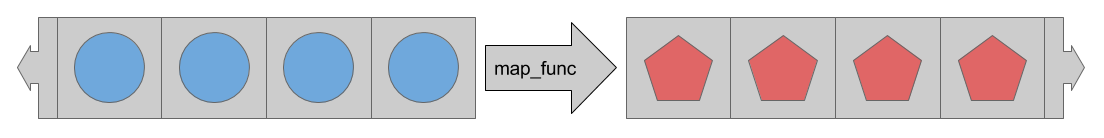Join us at TensorFlow World, Oct 28-31. Use code TF20 for 20% off select passes.

# Estimator 的数据集

tf.data 模块包含一系列类，可让您轻松地加载数据、操作数据并通过管道将数据传送到模型中。本文档通过两个简单的示例来介绍该 API：

• 从 Numpy 数组中读取内存中的数据。
• 从 csv 文件中读取行。

## 基本输入

def train_input_fn(features, labels, batch_size):
"""An input function for training"""
# Convert the inputs to a Dataset.
dataset = tf.data.Dataset.from_tensor_slices((dict(features), labels))

# Shuffle, repeat, and batch the examples.
dataset = dataset.shuffle(1000).repeat().batch(batch_size)

# Return the dataset.
return dataset


### 参数

• features：包含原始输入特征的 {'feature_name':array} 字典（或 DataFrame）。
• labels：包含每个样本的标签的数组。
• batch_size：表示所需批次大小的整数。

premade_estimator.py 中，我们使用 iris_data.load_data() 函数检索了鸢尾花数据。您可以运行该函数并解压结果，如下所示：

import iris_data

# Fetch the data
train, test = iris_data.load_data()
features, labels = train


batch_size=100
iris_data.train_input_fn(features, labels, batch_size)


### 切片

train, test = tf.keras.datasets.mnist.load_data()
mnist_x, mnist_y = train

mnist_ds = tf.data.Dataset.from_tensor_slices(mnist_x)
print(mnist_ds)


<TensorSliceDataset shapes: (28,28), types: tf.uint8>


dataset = tf.data.Dataset.from_tensor_slices(dict(features))
print(dataset)

<TensorSliceDataset

shapes: {
SepalLength: (), PetalWidth: (),
PetalLength: (), SepalWidth: ()},

types: {
SepalLength: tf.float64, PetalWidth: tf.float64,
PetalLength: tf.float64, SepalWidth: tf.float64}
>


# Convert the inputs to a Dataset.
dataset = tf.data.Dataset.from_tensor_slices((dict(features), labels))
print(dataset)

<TensorSliceDataset
shapes: (
{
SepalLength: (), PetalWidth: (),
PetalLength: (), SepalWidth: ()},
()),

types: (
{
SepalLength: tf.float64, PetalWidth: tf.float64,
PetalLength: tf.float64, SepalWidth: tf.float64},
tf.int64)>


### 操作

# Shuffle, repeat, and batch the examples.
dataset = dataset.shuffle(1000).repeat().batch(batch_size)


shuffle 方法使用一个固定大小的缓冲区，在条目经过时随机化处理条目。在这种情况下，buffer_size 大于 Dataset 中样本的数量，确保数据完全被随机化处理（鸢尾花数据集仅包含 150 个样本）。

repeat 方法会在结束时重启 Dataset。要限制周期数量，请设置 count 参数。

batch 方法会收集大量样本并将它们堆叠起来以创建批次。这为批次的形状增加了一个维度。新的维度将添加为第一个维度。以下代码对之前的 MNIST Dataset 使用 batch 方法。这样会产生一个包含表示 (28,28) 图像堆叠的三维数组的 Dataset

print(mnist_ds.batch(100))

<BatchDataset
shapes: (?, 28, 28),
types: tf.uint8>


train_input_fn 中，经过批处理之后，Dataset 包含元素的一维向量，其中每个标量之前如下所示：

print(dataset)

<TensorSliceDataset
shapes: (
{
SepalLength: (?,), PetalWidth: (?,),
PetalLength: (?,), SepalWidth: (?,)},
(?,)),

types: (
{
SepalLength: tf.float64, PetalWidth: tf.float64,
PetalLength: tf.float64, SepalWidth: tf.float64},
tf.int64)>


## 读取 CSV 文件

Dataset 类最常见的实际用例是流式传输磁盘上文件中的数据。tf.data 模块包含各种文件阅读器。我们来看看如何使用 Dataset 解析 csv 文件中的 Iris 数据集。

iris_data.maybe_download 函数的以下调用会根据需要下载数据，并返回所生成文件的路径名：

import iris_data


iris_data.csv_input_fn 函数包含使用 Dataset 解析 csv 文件的备用实现。

### 构建 Dataset

ds = tf.data.TextLineDataset(train_path).skip(1)


### 构建 csv 行解析器

# Metadata describing the text columns
COLUMNS = ['SepalLength', 'SepalWidth',
'PetalLength', 'PetalWidth',
'label']
FIELD_DEFAULTS = [[0.0], [0.0], [0.0], [0.0], ]
def _parse_line(line):
# Decode the line into its fields
fields = tf.decode_csv(line, FIELD_DEFAULTS)

# Pack the result into a dictionary
features = dict(zip(COLUMNS,fields))

# Separate the label from the features
label = features.pop('label')

return features, label


### 解析行

map 方法会接受 map_func 参数，此参数描述了应该如何转换 Dataset 中的每个条目。map 方法运用 map_func 来转换 Dataset 中的每个条目。

ds = ds.map(_parse_line)
print(ds)

<MapDataset
shapes: (
{SepalLength: (), PetalWidth: (), ...},
()),
types: (
{SepalLength: tf.float32, PetalWidth: tf.float32, ...},
tf.int32)>


iris_data.csv_input_fn 函数的剩余部分与 iris_data.train_input_fn 函数完全相同，后者在基本输入部分中进行了介绍。

### 试试看

train_path, test_path = iris_data.maybe_download()

# All the inputs are numeric
feature_columns = [
tf.feature_column.numeric_column(name)
for name in iris_data.CSV_COLUMN_NAMES[:-1]]

# Build the estimator
est = tf.estimator.LinearClassifier(feature_columns,
n_classes=3)
# Train the estimator
batch_size = 100
est.train(
steps=1000,
input_fn=lambda : iris_data.csv_input_fn(train_path, batch_size))


Estimator 要求 input_fn 不接受任何参数。为了不受此限制约束，我们使用 lambda 来获取参数并提供所需的接口。

## 总结

tf.data 模块提供一系列类和函数，可用于轻松从各种来源读取数据。此外，tf.data 还提供简单而又强大的方法，用于应用各种标准和自定义转换。

• 创建自定义 Estimator：展示了如何自行构建自定义 Estimator 模型。
• 低阶 API 简介：展示了如何使用 TensorFlow 的低阶 API 直接尝试 tf.data.Datasets
• 导入数据：详细介绍了 Datasets 的其他功能。